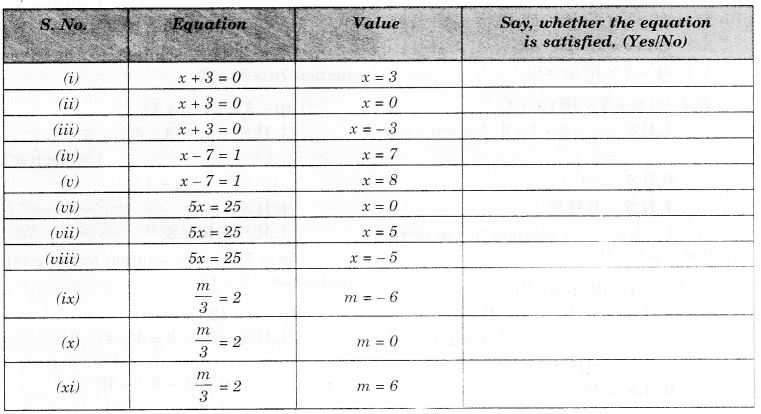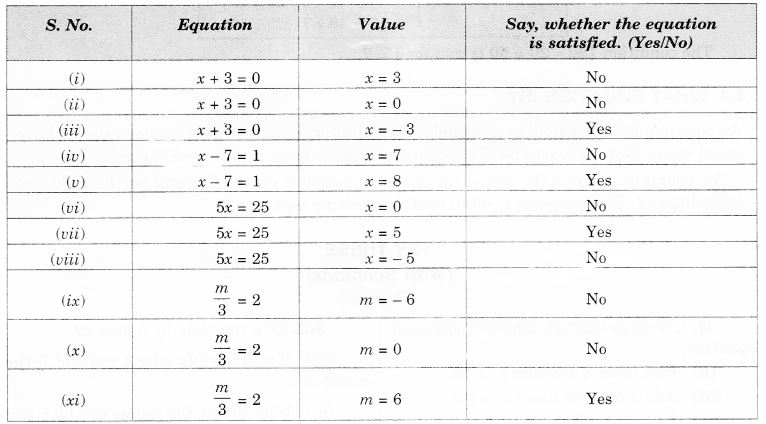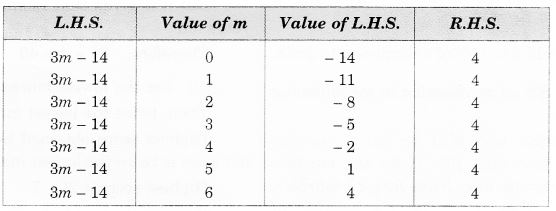NCERT Solutions for Class 7 Maths Chapter 4 Simple Equations Ex 4.1 are part of NCERT Solutions for Class 7 Maths. Here we have given NCERT Solutions for Class 7 Maths Chapter 4 Simple Equations Ex 4.1.

 Board CBSE Textbook NCERT Class Class 7 Subject Maths Chapter Chapter 4 Chapter Name Simple Equations Exercise Ex 4.1 Number of Questions Solved 6 Category NCERT Solutions

## NCERT Solutions for Class 7 Maths Chapter 4 Simple Equations Ex 4.1

Question 1.
Complete the last column of the table.Solution:Question 2.
Check whether the value given in the brackets is a solution to the given equation or not.

(a) n + 5 = 19 (n = 1)
(b) 7n + 5 = 19 (n = – 2)
(c) 7n + 5 = 19 (n = 2)
(d) 4p – 3 = 13 (p = 1)
(e) 4p – 3 = 13 (p = – 4)
(f) 4p – 3 = 13 (p = 0).

Solution:
(a) n + 5 = 19 (n = 1)
L.H.S. = n + 5 = 1 + 5 | when n = 1 = 5
R.H.S. = 19
∵ L.H.S. ≠ R.H.S.
∴ n = 1 is not a solution to the given equation n + 5 = 19.

(b) 7n + 5 = 19 (n = – 2)
L.H.S. = 7n + 5 = 7(- 2) + 5 | when n = – 2 = – 14 + 5 = – 9
R.H.S. = 19
∵ L.H.S. ≠ R.H.S.
∴ n = – 2 is not a solution to the given equation 7n + 5 = 19.

(c) 7n + 5 = 19 (n = 2)
L.H.S. = In + 5 = 7(2) + 5 | when n = 2 = 14 + 5 = 19 = R.H.S.
∴ n = 2 is a solution to the given equation 7n + 5 = 19.

(d) 4p – 3 = 13 (p = 1)
L.H.S. = 4p – 3 = 4(1) – 3 | when p = 1 = 4 – 3 = 1
R.H.S. = 13
∵ L.H.S. ≠ R.H.S.
∴ p = 1 is not a solution to the given equation 4p – 3 = 13.

(e) 4p – 3 = 13 (p = – 4)
L.H.S. = 4p – 3 = 4(- 4) – 3 , | when p = – 4 = – 16 – 3 = – 19
R.H.S. = 13
∵ L.H.S. ≠ R.H.S.
∴ p = – 4 is not a solution to the given equation
4p – 3 = 13.

(f) 4p – 3 = 13 (p = 0)
L.H.S. = 4 (p) – 3 = 4(0) – 3 | when p = 0 = 0 – 3 = – 3
R.H.S. = 13
∵ L.H.S. ≠ R.H.S.
∴ p = 0 is not a solution to the given equation 4p – 3 = 13.

Question 3.
Solve the following equations by trial and error method.

1. 5p + 2 = 17
2. 3m – 14 = 4.

Solution:
(i) 5p + 2 = 17So, p = 3 is the solution of the given equation 5p + 2 = 17.

(ii) 3m – 14 = 4So, m = 6 is the solution of the given equation 3m – 14 = 4.

Question 4.
Write equations for the following statements.

1. The sum of numbers x and 4 is 9.
2. 2 subtracted from y is 8.
3. Ten times a is 70.
4. The number b divided by 5 gives 6.
5. Three-fourth oft is 15.
6. Seven times m plus 7 gets you 77.
7. One-fourth of a number x minus 4 gives 4.
8. If you take away 6 from 6 times y, you get 60.
9. If you add 3 to one-third of z, you get 30.

Solution:

1. x + 4 = 9
2. y – 2 = 8
3. 10 a = 70
4. b ÷ 5 = 6
5. $$\frac { 3 }{ 4 }$$ × t = 15
6. 7m + 7 = 77
7. $$\frac { 1 }{ 4 }$$ × x – 4 = 4
8. 6y – 6 = 60
9. $$\frac { 1 }{ 3 }$$ × z + 3 = 30

Question 5.
Write the following equations in statement forms:

1. p + 4 = 15
2. m – 7 = 3
3. 2m = 7
4. $$\frac { m }{ 5 }$$ = 3
5. $$\frac { 3m }{ 5 }$$ = 6
6. 3p + 4 = 25
7. 4p – 2 = 18
8. $$\frac { p }{ 2 }$$ + 2 = 8.

Solution:

1. The sum of p and 4 is 15.
2. 7 subtracted from m is 3.
3. Twice a number m is 7.
4. One-fifth of a number m is 3.
5. Three-fifth of a number m is 6.
6. Three times a number p, when added to 4, gives 25.
7. 2 subtracted from four times a number p is 18.
8. Add 2 to half of a number p to get 8.

Question 6.
Set up an equation in the following cases:

1. Irfan says that he has 7 marbles more than five times the marbles Permit has. Irfan has 37 marbles. (Take m to be the number of Permit’s marbles.)
2. Laxmi’s father is 49 years old. He is 4 years older than three times Laxmi’s age. (Take Laxmi’s age to be y years.)
3. The teacher tells the class that the highest marks obtained by a student in her class are twice the lowest marks plus 7. The highest score is 87. (Take the lowest score to be l.)
4. In an isosceles triangle, the vertex angle is twice either base angle. (Let the base angle be b in degrees. Remember that the sum of angles of triangle is 180 degrees).

Solution:
(i) Let the number of marbles with Parmit be m.
Then, 7 added to 5 times mis 5m + 7
It is given that 7 marbles more than five times the marble is 37. Thus, the equation obtained is 5m + 7 = 37.

(ii) Let Laxmi’s age be y years. Then, 4 added to 3 times y is 3y + 4
It is given that the father is 4 years older than 3 times Laxmi’s age. His age is 49years.
Then, we have the following equation : 3y + 4 = 49

(iii) Let the lowest marks be l. Then, twice the lowest marks plus 7 is 2l +7
It is given that, the highest marks 87 obtained by a student is twice the lowest marks plus 7.
So, we have the following equation : 2l + 7 = 87

(iv) Let the base angle be b. Then, the vertex angle = 2b.
Since, sum of the angles of a triangle is 180°
∴ b + b + 2b = 180°
⇒ 4b = 180°
which is the required equation.

We hope the NCERT Solutions for Class 7 Maths Chapter 4 Simple Equations Ex 4.1 help you. If you have any query regarding NCERT Solutions for Class 7 Maths Chapter 4 Simple Equations Ex 4.1, drop a comment below and we will get back to you at the earliest.